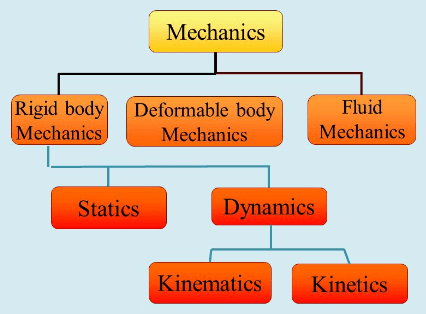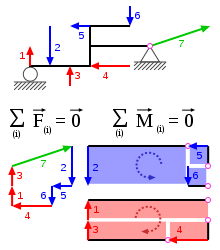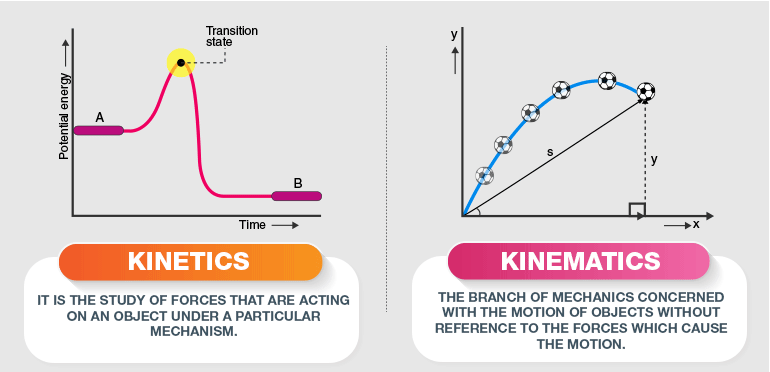Basics of Mechanics

# Basics of Mechanics - Notes | Study Engineering Mechanics - Mechanical Engineering

 Table of contentsIntroductionStaticsDynamics1 Crore+ students have signed up on EduRev. Have you?

Introduction

• From the very childhood, we have seen footballs bouncing and wheels rolling. We might have wondered how all these motions happen. These all motions are the interaction of different bodies and the effect of forces acting on them
• The branch of science dealing with the effect of forces on bodies is called Mechanics. The principles of Mechanics are exactly applicable to machines, it may be a simple machine such as a liver or bicycle or a machine as complex as an aircraft.• When Mechanics is applied in Engineering, design, and analysis of mechanisms and machines, it is known as Engineering Mechanics.
• When bodies interact and forces act between them there are two possibilities, they may move or they may remain static. The branch of Engineering Mechanics dealing with the motion of bodies is known as Dynamics and the other branch is known as Statics, in which we study the balance and equilibrium of bodies.
• Throughout the study of Engineering Mechanics, the principles of three Newton's Laws of Motion are used invariably. So complete understanding of these laws is a must.
• Along with this, as the physical quantities encountered during engineering analyses are mostly vectors, adequate knowledge of vector algebra is required.
Statics
• This branch of engineering mechanics deals with bodies in equilibrium and are not moving with respect to the frame of reference considered for analysis.
• Bodies may be experiencing different forces but the configuration of these forces is such that the resultant force on the system is zero. The unbalanced forces tend to accelerate a body but if the net force is zero the body will not accelerate.
• In addition to accelerating a body forces make bodies rotate. This ability of a force to rotate a body is known as torque or moment of the force
• For true static equilibrium, the net moment or torque on a body should also be zero along with zero net force.• Statics include force analysis in stationary structures such as trusses, frames, and machines at certain stationary positions. Cables and strings in stationary positions in mechanical systems also come under the purview of statics.

Question for Basics of Mechanics
Try yourself:In Theory of machines ____ deals with various forces when the body is stationary.

Dynamics
• The analysis of forces and motion in moving bodies comes in Dynamics.
• This branch of engineering mechanics is further divided into two branches:
(i) Kinematics deals with the analysis of the motion of bodies without considering the forces causing or associated with these motions. In Kinematics the position, velocity, and acceleration of certain points and the members of mechanisms and machines are studied. The kinematic analysis starts with particles and is then extended to rigid bodies.(ii)  Kinetics includes analysis of causal force, impulse, and momentum of bodies. The forces causing motion in bodies are studied under kinetics. The concept of work and energy and its application for the analysis of mechanical systems also comes in this branch of dynamics.

Question for Basics of Mechanics
Try yourself:______ is the study to know the displacement, velocity and acceleration of a part of the machine.

The document Basics of Mechanics - Notes | Study Engineering Mechanics - Mechanical Engineering is a part of the Mechanical Engineering Course Engineering Mechanics.
All you need of Mechanical Engineering at this link: Mechanical Engineering

## Engineering Mechanics

24 videos|71 docs|58 tests
 Use Code STAYHOME200 and get INR 200 additional OFF

## Engineering Mechanics

24 videos|71 docs|58 tests

Track your progress, build streaks, highlight & save important lessons and more!

,

,

,

,

,

,

,

,

,

,

,

,

,

,

,

,

,

,

,

,

,

;